# Fractions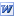Fraction Workout Equivalent fractions, simplifying, multiplying, Top heavy to mixed, dividing, mixed to top heavy, fractions of, fractions to percentages, adding & subtracting. 10 questions on each. Nice! Pdf here.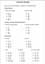Fractions Checkup Word doc of various fraction questions (equivalent, top heavy, add subtract, multiply, divide). Includes answers. PDF here.Fraction Notes Crib sheet on how to cancel fractions down, multiply, divide, add and subtract fractions as well as converting betwen mixed numbers and top heavy fractions.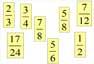Fractions Order Arrange the fractions in order, smallest at the back, largest at the front.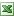Fraction Image MS Excel program to generate areas (upto 10x10) to demonstrate fractions and to explain, for example, how come 3/6 is the same as 1/2 etc.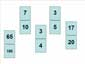Fractions This is created as an example of how an interactive whiteboard can be used interactively with pupils. Put the fractions in ascending order (lowest values at bottom of pile).Fractions II Put the fractions in ascending order (highest values at bottom of pile). If pupils need help then there are equivalent fractions hidden underneath the white rectangle (delete the rectangle to reveal).